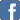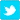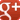# Fun with Numbers

This was explained to me a decade ago (by an Indian granddad in the playground when we were picking up kids from school), but I forgot how to do it, so looked it up again a couple of days ago.
Here's how we were taught to do long multiplication at primary school. Makes sense, is methodical, but is difficult to do in your head, remembering the hundreds, tens and units columns:Vedic multiplication requires far fewer digits in the workings. You start by multiplying the first two (5, 4) and writing 20 so that the last digit 0 lines up with the 5 and the 4. Then you add 5x3 and 4x2 = 23, write the last digit 3 one column to the left of the 20 etc. The only tricky bit is to remember that the last digit of 2 x 3 lines up with the last digit of 5x9 plus 4x1 = 23, because 'tens x tens' is the same or of magnitude as 'hundreds x units':Not only is it easier on paper, it lends itself to mental arithmetic. You start with 20, it doesn't matter that this actually represents 200,000. Then you multiply it by ten and add 5x3 and 4x2, and so on. You just keep a running tally. Depending on what level of accuracy you want. You could just go as far as 2,279, round it up 'a bit' and then multiply by a hundred (you know that the answer is going to be 'a bit more than' 500 x 400 = 200,000):